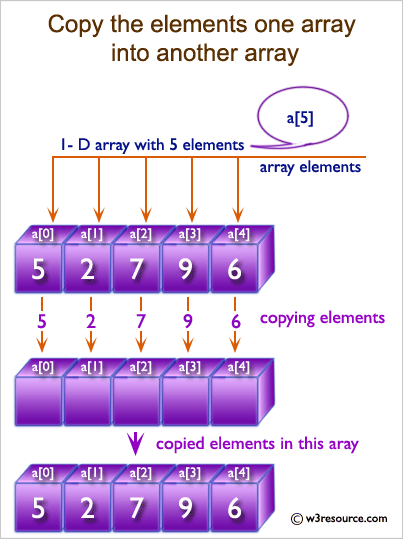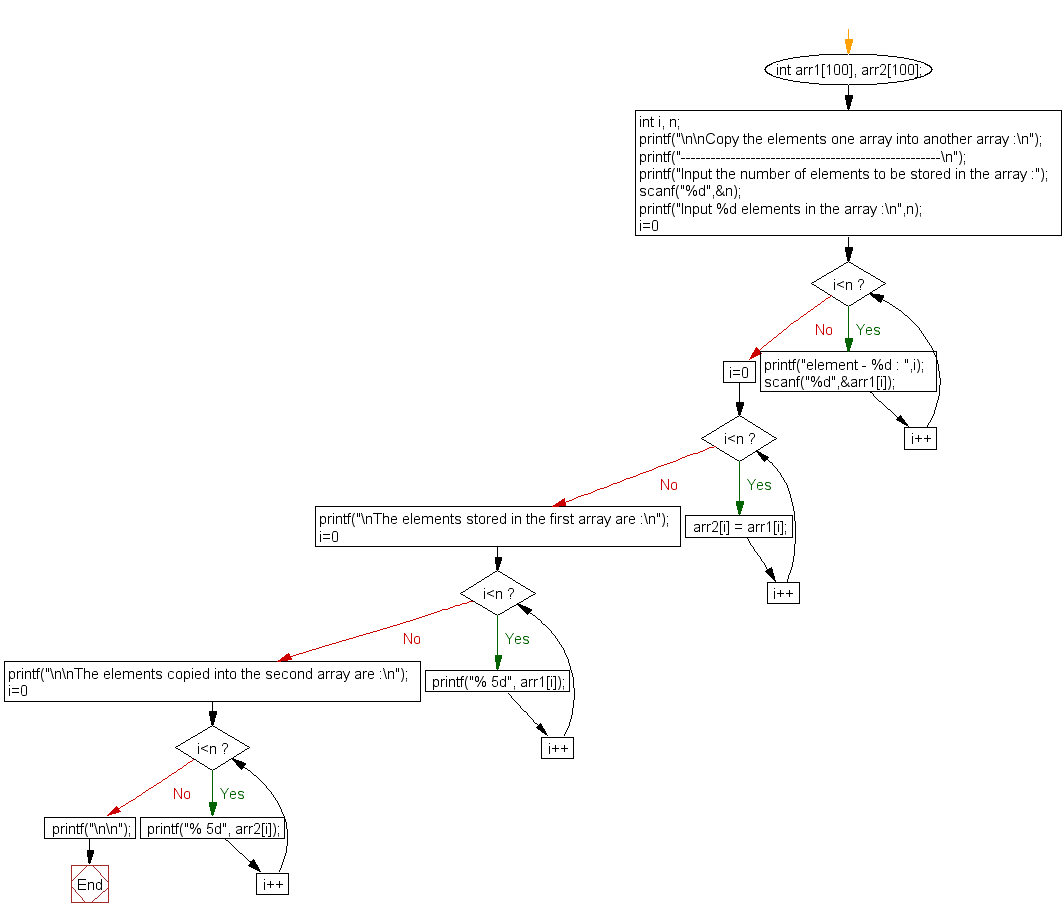﻿ C Program: Copy the elements of one array into another array - w3resource

# C Exercises: Copy the elements of one array into another array

## C Array: Exercise-4 with Solution

Write a program in C to copy the elements of one array into another array.

Visual Presentation:Sample Solution:

C Code:

``````#include <stdio.h>
// Main function
int main()
{
int arr1, arr2;  // Declare two arrays of size 100 to store integer values
int i, n;  // Declare variables to store array size and loop counter
// Display a message to the user about the program's purpose
printf("\n\nCopy the elements of one array into another array:\n");
printf("----------------------------------------------------\n");
// Prompt the user to input the number of elements to be stored in the array
printf("Input the number of elements to be stored in the array :");
scanf("%d", &n);
// Prompt the user to input n elements into the first array
printf("Input %d elements in the array :\n", n);
for (i = 0; i < n; i++)
{
printf("element - %d : ", i);
scanf("%d", &arr1[i]);  // Read the input and store it in the first array
}
/* Copy elements of the first array into the second array. */
for (i = 0; i < n; i++)
{
arr2[i] = arr1[i];  // Copy each element from the first array to the second array
}

/* Prints the elements of the first array */
printf("\nThe elements stored in the first array are :\n");
for (i = 0; i < n; i++)
{
printf("% 5d", arr1[i]);  // Print each element in the first array
}
/* Prints the elements copied into the second array. */
printf("\n\nThe elements copied into the second array are :\n");
for (i = 0; i < n; i++)
{
printf("% 5d", arr2[i]);  // Print each element in the second array
}
printf("\n\n");  // Print new lines for better formatting
return 0;
}
```
```

Sample Output:

```Copy the elements one array into another array :
----------------------------------------------------
Input the number of elements to be stored in the array :3
Input 3 elements in the array :
element - 0 : 15
element - 1 : 10
element - 2 : 12

The elements stored in the first array are :
15   10   12

The elements copied into the second array are :
15   10   12
```

Explanation:

``` printf("Input the number of elements to be stored in the array :");
scanf("%d",&n);

printf("Input %d elements in the array :\n",n);
for(i=0;i<n;i++)
{
printf("element - %d : ",i);
scanf("%d",&arr1[i]);
}
/* Copy elements of first array into second array.*/
for(i=0; i<n; i++)
{
arr2[i] = arr1[i];
}

/* Prints the elements of first array   */
printf("\nThe elements stored in the first array are :\n");
for(i=0; i<n; i++)
{
printf("% 5d", arr1[i]);
}

/* Prints the elements copied into the second array. */
printf("\n\nThe elements copied into the second array are :\n");
for(i=0; i<n; i++)
{
printf("% 5d", arr2[i]);
}
printf("\n\n");
```

In the above code -

• The first printf statement prompts the user to input the number of elements they want to store in the array and stores it in the variable n using scanf.
• The second printf statement asks the user to input n number of elements into the first array arr1 using a for loop, and stores each input in the corresponding index of the array arr1[i].
• The third for loop then copies the elements of arr1 into a second array arr2 by iterating over each element of arr1 and assigning it to the corresponding index of arr2.
• The next two printf statements then print out the contents of the first array arr1 and the second array arr2 respectively, using separate for loops to iterate over the elements of each array and print them out using printf.

Flowchart:C Programming Code Editor:

What is the difficulty level of this exercise?

Test your Programming skills with w3resource's quiz.

﻿